# Angle Sum Property of a Triangle & Exterior Angle Property Notes | Study Mathematics (Maths) Class 9 - Class 9

## Class 9: Angle Sum Property of a Triangle & Exterior Angle Property Notes | Study Mathematics (Maths) Class 9 - Class 9

The document Angle Sum Property of a Triangle & Exterior Angle Property Notes | Study Mathematics (Maths) Class 9 - Class 9 is a part of the Class 9 Course Mathematics (Maths) Class 9.
All you need of Class 9 at this link: Class 9

Angle Sum Property of a Triangle Theorem

In the given triangle, ∆ABC, AB, BC, and CA represent three sides. A, B and C are the three vertices and ∠ABC, ∠BCA and ∠CAB are three interior angles of ∆ABC.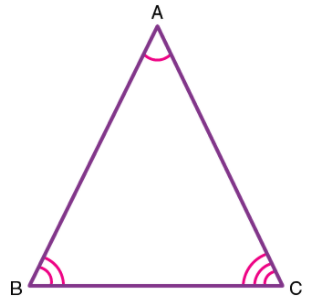Theorem 1: Angle sum property of triangle states that the sum of interior angles of a triangle is 180°.
Proof:
Consider a ∆ABC, as shown in the figure below. To prove the above property of triangles, draw a line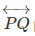parallel to the side BC of the given triangle.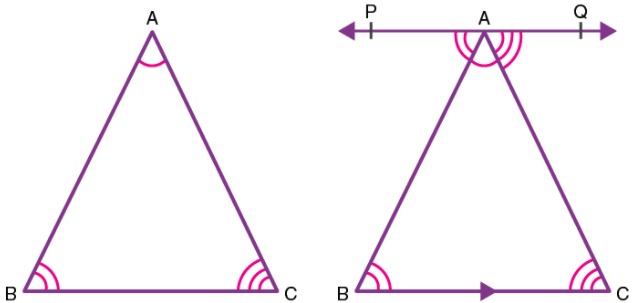Since PQ is a straight line, it can be concluded that:
∠PAB + ∠BAC + ∠QAC = 180°  ………(1)
Since PQ||BC and AB, AC are transversals,
Therefore, ∠QAC = ∠ACB (a pair of alternate angle)
Also, ∠PAB = ∠CBA (a pair of alternate angle)
Substituting the value of ∠QAC and ∠PAB in equation (1),
∠ACB + ∠BAC + ∠CBA= 180°
Thus, the sum of the interior angles of a triangle is 180°.

Exterior Angle Property of a Triangle Theorem

Theorem 2: If any side of a triangle is extended, then the exterior angle so formed is the sum of the two opposite interior angles of the triangle.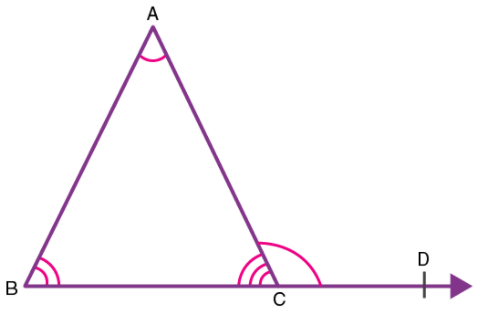In the given figure, the side BC of ∆ABC is extended. The exterior angle ∠ACD so formed is the sum of measures of ∠ABC and ∠CAB.

Proof:
From figure 3, ∠ACB and ∠ACD form a linear pair since they represent the adjacent angles on a straight line.
Thus, ∠ACB + ∠ACD = 180°  ……….(2)
Also, from the angle sum property, it follows that:
∠ACB + ∠BAC + ∠CBA = 180° ……….(3)
From equation (2) and (3) it follows that:
∠ACD = ∠BAC + ∠CBA
This property can also be proved using the concept of parallel lines as follows: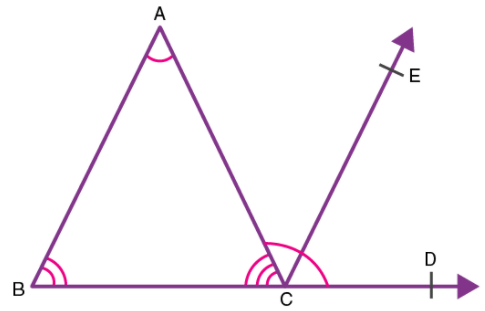In the given figure, side BC of ∆ABC is extended. A line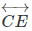parallel to the side AB is drawn, then: Since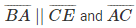is the transversal,

∠CAB = ∠ACE   ………(4) (Pair of alternate angles)
Also,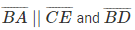is the transversal
Therefore, ∠ABC = ∠ECD  ……….(5) (Corresponding angles)
We have, ∠ACB + ∠BAC + ∠CBA = 180° ………(6)
Since the sum of angles on a straight line is 180°
Therefore, ∠ACB + ∠ACE + ∠ECD = 180° ………(7)
Since, ∠ACE + ∠ECD = ∠ACD(From figure 4)
Substituting this value in equation (7);
∠ACB + ∠ACD = 180° ………(8)
From the equations (6) and (8) it follows that,
∠ACD = ∠BAC + ∠CBA
Hence, it can be seen that the exterior angle of a triangle equals the sum of its opposite interior angles.

The document Angle Sum Property of a Triangle & Exterior Angle Property Notes | Study Mathematics (Maths) Class 9 - Class 9 is a part of the Class 9 Course Mathematics (Maths) Class 9.
All you need of Class 9 at this link: Class 9Use Code STAYHOME200 and get INR 200 additional OFF

## Mathematics (Maths) Class 9

84 videos|352 docs|109 tests

Track your progress, build streaks, highlight & save important lessons and more!

,

,

,

,

,

,

,

,

,

,

,

,

,

,

,

,

,

,

,

,

,

;